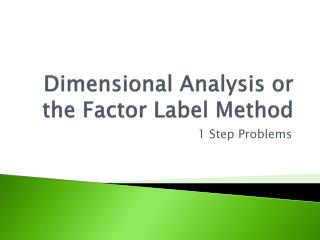Download PresentationDimensional Analysis or the Factor Label Method

# Dimensional Analysis or the Factor Label Method - PowerPoint PPT PresentationDownload Presentation## Dimensional Analysis or the Factor Label Method

- - - - - - - - - - - - - - - - - - - - - - - - - - - E N D - - - - - - - - - - - - - - - - - - - - - - - - - - -
##### Presentation Transcript

1. Dimensional Analysis or the Factor Label Method 1 Step Problems

2. Base Units Base Unit – a unit of measurement that does not have a prefix Time – second (s) Length – meter (m) Mass – gram (g) Volume – liter (L)

3. Metric Prefixes • Prefix – precedes a base unit of measure to indicate a multiple or a fraction of the unit • Each prefix has a unique symbol that is added to the beginning of the base unit • Example: the prefix kilo- has a symbol of k- the base unit meter has a symbol of m add the prefix to the base unit: kilometer, km

4. Larger than the base unit Mega- (M) 1 x 106 g = 1 Mg means 1,000,000 Kilo- (k) 1 x 103 L = 1 kL means 1,000 Hecto- (h) 1 x 102 m = 1 hm means 100 Deca- (da) 1 x 101 g = 1 dag means 10

5. Smaller than the base unit deci- (d) 1 g = 1 x 101 dg means 1/10 centi- (c) 1 L = 1 x 102cL means 1/100 milli- (m) 1 m = 1 x 103 mm means 1/1,000

6. Smaller than the base unit micro- () 1 g = 1 x 106g means 1/1,000,000 nano- (n) 1 L = 1 x 109nL means 1/1,000,000,000 Angstrom (Å) 1 m = 1 x 1010Å means 1/10,000,000,000 pico- (p) 1 g = 1 x 1012pg means 1/1,000,000,000,000

7. Dimensional Analysis or the Factor Label Method 1 step problem Example – How many grams are in 3.42 kg? • Write down your known 3.42 kg

8. 1 Step Problem Example – How many grams are in 3.42 kg? • Write down the conversion that involves your known unit on bottom and the unit you want to go to on top. 3.42 kg 1000 g 1 kg

9. 1 Step Problem You can either write the conversion in a “ladder” 3.42 kg 1000 g 1 kg Or as a multiplication factor: 3.42 kg x 1000 g 1 kg

10. 1 Step Problem 3. Cancel out units and solve. • Remember if you have the same unit on top and on bottom, they cancel out. 3.42 kg x 1000 g =3420 g 1 kg

11. Practice How many Liters are in 1.5 x 106nL? = 1.5 x 10-3 L

12. Practice How many milligrams are in 260 grams? = 260,000 mg